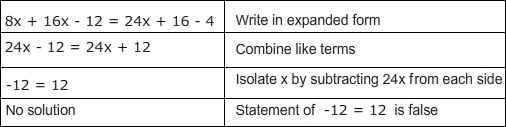# Solving Linear Equations : Solving Linear Equations Quiz

Quiz
*Theme/Title: Solving Linear Equations
* Description/Instructions
A linear equation is an algebraic equation whose solutions form a linear graph. When solving for a variable, equations will either have one solution, no solution, or infinite solutions. For example, x = 3 is one solution, 0 = 3 is no solution (a false statement), and 3 = 3 is infinite solutions (a true statement without variable). Keeping these possibilities in mind, solve for the variable in the following equations.

Example: 8x + 4(4x - 3) = 4(6x + 4) - 4Group: Algebra Algebra Quizzes
Topic: Solving Linear Equations